Examples

Chapter 7 Class 10 Coordinate Geometry
Serial order wise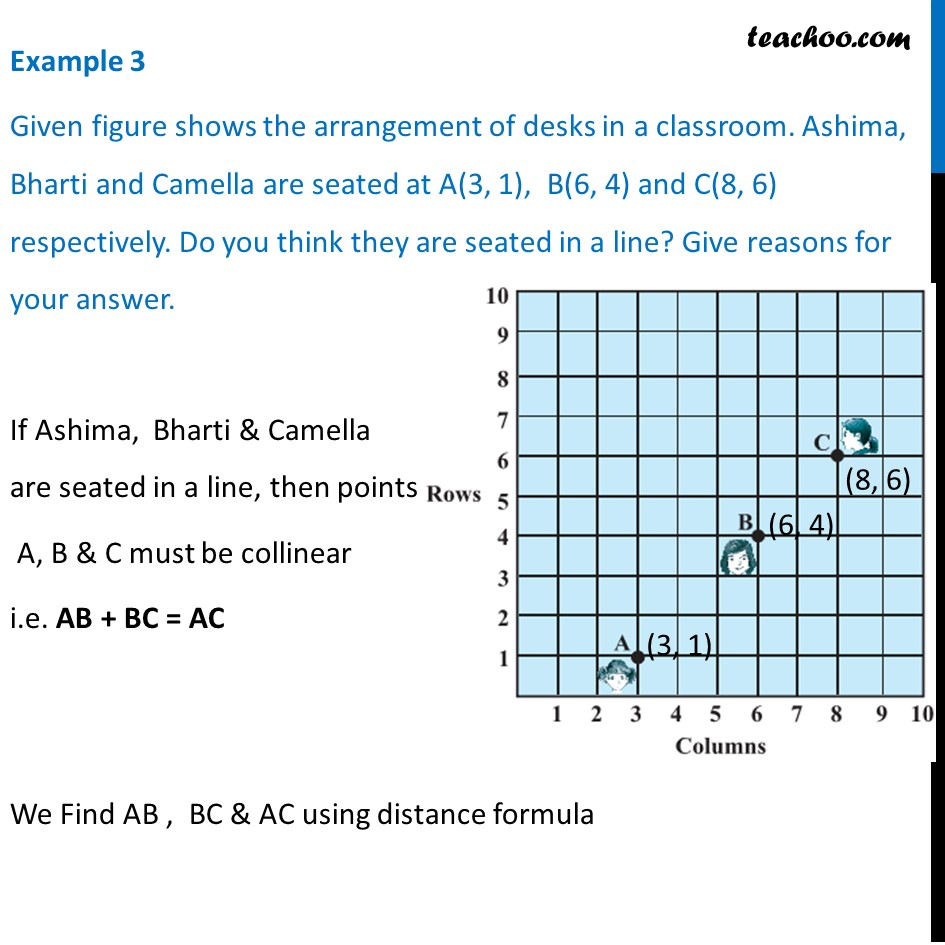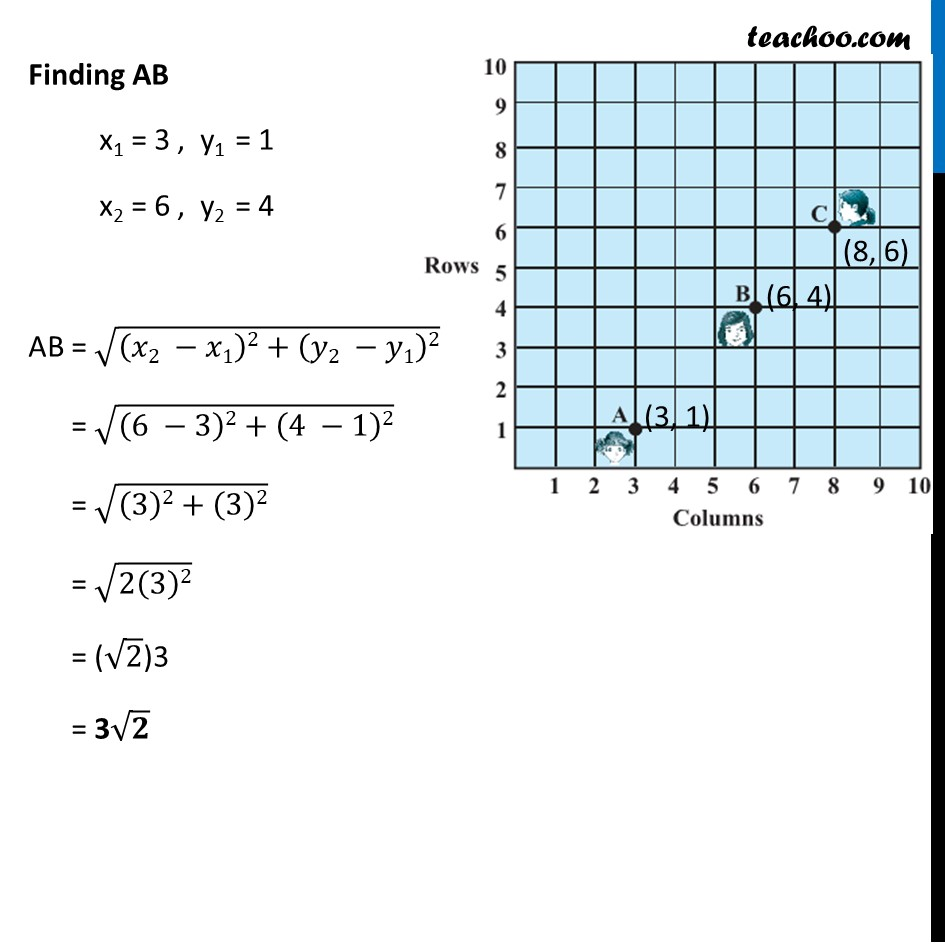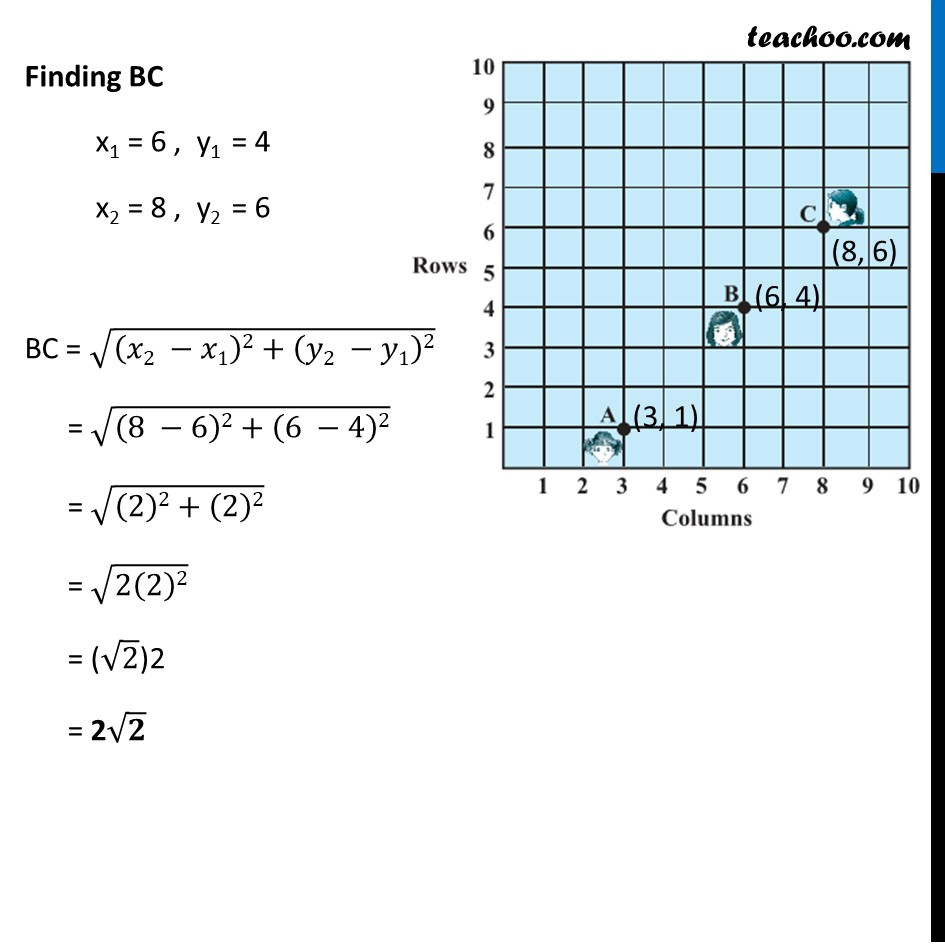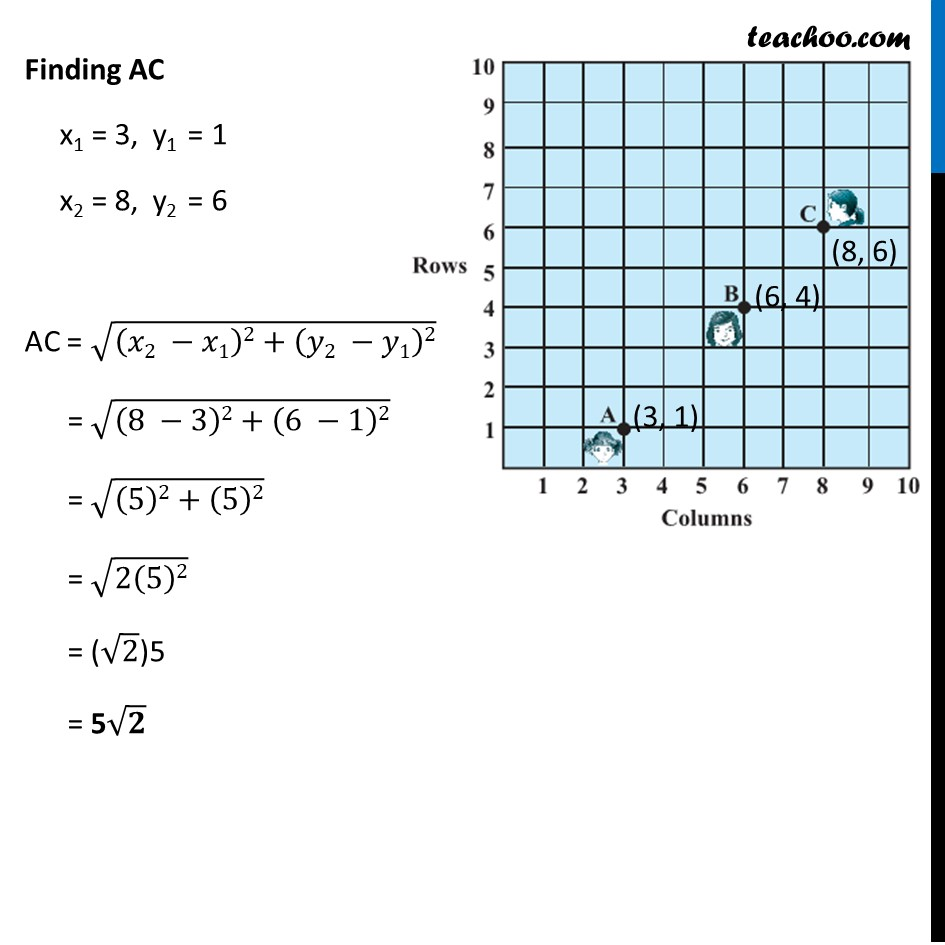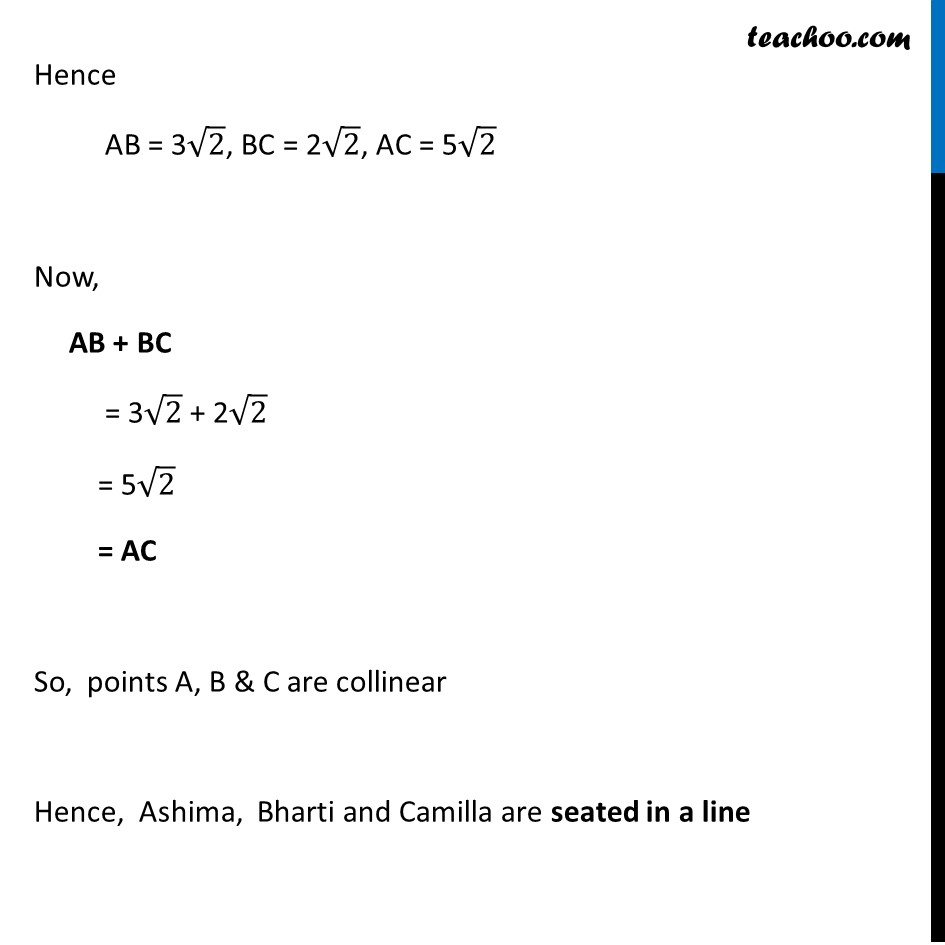Learn in your speed, with individual attention - Teachoo Maths 1-on-1 Class

### Transcript

Example 3 Given figure shows the arrangement of desks in a classroom. Ashima, Bharti and Camella are seated at A(3, 1), B(6, 4) and C(8, 6) respectively. Do you think they are seated in a line? Give reasons for your answer. If Ashima, Bharti & Camella are seated in a line, then points A, B & C must be collinear i.e. AB + BC = AC We Find AB , BC & AC using distance formula Finding AB x1 = 3 , y1 = 1 x2 = 6 , y2 = 4 AB = √((𝑥2 −𝑥1)2+(𝑦2 −𝑦1)2) = √((6 −3)2+(4 −1)2) = √((3)2+(3)2) = √(2(3)2) = (√2)3 = 3√𝟐 Finding BC x1 = 6 , y1 = 4 x2 = 8 , y2 = 6 BC = √((𝑥2 −𝑥1)2+(𝑦2 −𝑦1)2) = √((8 −6)2+(6 −4)2) = √((2)2+(2)2) = √(2(2)2) = (√2)2 = 2√𝟐 Finding AC x1 = 3, y1 = 1 x2 = 8, y2 = 6 AC = √((𝑥2 −𝑥1)2+(𝑦2 −𝑦1)2) = √((8 −3)2+(6 −1)2) = √((5)2+(5)2) = √(2(5)2) = (√2)5 = 5√𝟐 Hence AB = 3√2, BC = 2√2, AC = 5√2 Now, AB + BC = 3√2 + 2√2 = 5√2 = AC So, points A, B & C are collinear Hence, Ashima, Bharti and Camilla are seated in a line